# 4 Lines

The (cartesian) equation of a straight line is linear in the coordinates x and y, that is, of the form ax+by+c=0.

The slope of this line is -a/b, the intersection with the x-axis (or x-intercept) is x=-c/a, and the intersection with the y-axis (or y-intercept) is y=-c/b. If a=0 the line is parallel to the x-axis, and if b=0 the line is parallel to the y-axis.

(In an oblique coordinate system everything in the preceding paragraph remains true, except for the value of the slope.)

When a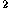+b=1 and c0 in the equation ax+by+c=0, the equation is said to be in normal form. In this case c is the distance of the line to the origin, and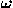= arcsin a = arccos b

is the angle that the perpendicular dropped to the line from the origin makes with the positive x-axis (Figure 1).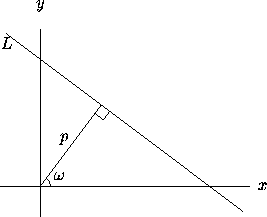Figure 1: The normal form of L is x cos+ y sin=p.

To reduce an arbitrary equation ax+by+c=0 to normal form, divide by ±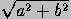, where the sign of the radical is chosen opposite the sign of c when c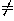0 and the same as the sign of b when c=0.

### Lines with prescribed properties

Line of slope m intersecting the x-axis at x=x:

y=m(x-x)

Line of slope m intersecting the y-axis at y=y:

y=mx+yLine intersecting the x-axis at x=xand the y-axis at y=y:

x/x+y/y=1

(This formula remains true in oblique coordinates.)

Line of slope m going though (x,y):

y-y=m(x-x)

Line going through points (x,y) and (x,y):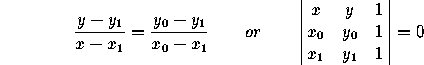(These formulas remain true in oblique coordinates.)

Slope of line going through points (x,y) and (x,y):

(y-y)/(x-x)

Line going through points with polar coordinates (r,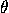) and (r,):

r(rsin(-)-rsin(-))= rrsin(-).

Next: 4.1 Distances
Up: Part I: Two-Dimensional Geometry
Previous: 3.3 Projective TransformationsThe Geometry Center Home Page

Silvio Levy
Wed Oct 4 16:41:25 PDT 1995

This document is excerpted from the 30th Edition of the CRC Standard Mathematical Tables and Formulas (CRC Press). Unauthorized duplication is forbidden.